• 如何判断系统为线性系统
千次阅读
2021-10-23 15:26:58

目前只写了叠加性部分，齐次性、定常时变类似（之后补）

如果不是微分方程中含有积分，那么两边微分/求导消去积分

# 线性系统

线性系统有两个重要的特性：叠加性和齐次性。

## 叠加性

### 定义

当系统同时存在几个输入量时，其输出量等于各输入量单独作用时所引起的输出量的和。
即当 r ( t ) = ∑ r i ( t ) r(t)=\sum{r_i(t)} 时，微分方程的解为 c ( t ) = ∑ c i ( t ) c(t)=\sum{c_i(t)} ，这就是叠加性。

### 判断方法

• 设各输入量 c i ( t ) c_i(t) 分别代入微分方程中时，输出量分别为 r i ( t ) r_i(t) （证明的时候取两个就可以了）
• 将各分量的微分方程相加，不管是否线性，等式都成立（式一）
• 将输入 c ( t ) = ∑ c i ( t ) c(t)=\sum{c_i(t)} 代入微分方程，如果满足叠加性，其输出量应为单独作用时所引起的输出量的和，即同时将 r ( t ) = ∑ r i ( t ) r(t)=\sum{r_i(t)} 代入微分方程中，等式应成立（式二）

式一是一定成立的，用于证明或者证伪式二。若能证明式二成立，则说明该系统满足叠加性，反之不满足。

#### 例题

例1：c(t)为输入，r(t)为输出，系统的微分方程为 t d c ( t ) d t + c ( t ) = r ( t ) + 3 d r ( t ) d t t\frac{dc(t)}{dt}+c(t)=r(t)+3\frac{dr(t)}{dt} .
解: 假设两个输入量 c 1 ( t ) , c 2 ( t ) c_1(t),c_2(t) 分别作用于系统，则由系统的微分方程，分别有
t d c 1 ( t ) d t + c 1 ( t ) = r 1 ( t ) + 3 d r 1 ( t ) d t    ① t\frac{dc_1(t)}{dt}+c_1(t)=r_1(t)+3\frac{dr_1(t)}{dt} \ \ ①
t d c 2 ( t ) d t + c 2 ( t ) = r 2 ( t ) + 3 d r 2 ( t ) d t    ② t\frac{dc_2(t)}{dt}+c_2(t)=r_2(t)+3\frac{dr_2(t)}{dt} \ \ ②
c 1 ( t ) + c 2 ( t ) c_1(t)+c_2(t) 同时作用于系统时，假设系统满足叠加性，应有
t d ( c 1 ( t ) + c 2 ( t ) ) d t + c 1 ( t ) + c 2 ( t ) = r 1 ( t ) + r 2 ( t ) + 3 d ( r 1 ( t ) + r 2 ( t ) ) d t    ③ ( 式 二 ) t\frac{d(c_1(t)+c_2(t))}{dt}+c_1(t)+c_2(t)=r_1(t)+r_2(t)+3\frac{d(r_1(t)+r_2(t))}{dt} \ \ ③(式二)
①+②得
t d ( c 1 ( t ) + c 2 ( t ) ) d t + c 1 ( t ) + c 2 ( t ) = r 1 ( t ) + r 2 ( t ) + 3 d ( r 1 ( t ) + r 2 ( t ) ) d t    ④ ( 式 一 ) t\frac{d(c_1(t)+c_2(t))}{dt}+c_1(t)+c_2(t)=r_1(t)+r_2(t)+3\frac{d(r_1(t)+r_2(t))}{dt} \ \ ④(式一)
可见③(式二)成立，所以假设成立，系统满足叠加性

若不满足叠加性，结论如下
可见④与③矛盾，故系统不满足叠加性

## 齐次性

### 定义

当输入量增大或缩小k (k为实数)倍时，系统输出量也按同一倍数增大或缩小。
即当 r ( t ) = k r 1 ( t ) r(t)=kr_1(t) 时(k为常数实数)，微分方程的解为 c ( t ) = k c 1 ( t ) c(t)=kc_1(t) .

还没写

还没写

# 非线性系统

在构成系统的环节中有一个或一个以上的非线性环节时，则称此系统为非线性系统。典型的非线性特性有饱和特性、死区特性、间隙特性、继电特性、磁滞特性等。

# 定常系统

如果系统中参数不随时间变化，则这类系统称为定常系统。在实践中遇到的系统，大多数属于这一类。

## 线性定常系统

如果一个线性系统微分方程的系数为常数，
那么系统称为线性定常系统。
例如：
d 2 c ( t ) d t 2 + 2 d c ( t ) d t + x ( t ) = r ( t ) \frac{d^2c(t)}{dt^2}+2\frac{dc(t)}{dt}+x(t)=r(t)

# 时变系统

如果系统中的参数是时间t的函数，则这类系统称为时变系统。

## 线性时变系统

如果一个线性系统微分方程的系数为时间的函数，
那么系统称为线性时变系统。
例如：
d 2 c ( t ) d t 2 + 2 t d c ( t ) d t + x ( t ) = r ( t ) \frac{d^2c(t)}{dt^2}+2t\frac{dc(t)}{dt}+x(t)=r(t)

参考老师上课内容以及网上课件https://www.docin.com/p-107264184.html

如果觉得我写的内容太烂，请点个踩或者评论和“垃圾”，或者说出改进的点数学
更多相关内容
• 　线性系统：状态变量和输出变量对于所有可能的输入变量和初始状态都满足叠加原理的系统。一个由线性元部件所组成的系统必是线性系统。但是，相反的命题在某些情况下可能不成立。线性系统的状态变量（或输出变量）与...
• 如何判断一个系统是否为线性系统，时不变系统以及稳定系统？先线性运算再经过系统=先经过系统再线性运算是线性系统；先时移再经过系统=先经过系统再时移为时不变系统；时间趋于无穷大时系统值有界则稳定的系统，...

如何判断一个系统是否为线性系统，时不变系统以及稳定系统？

先线性运算再经过系统=先经过系统再线性运算是线性系统；

先时移再经过系统=先经过系统再时移为时不变系统；

时间趋于无穷大时系统值有界则为稳定的系统，或者对连续系统S域变换，离散系统Z域变换，H(s)极点均在左半平面则稳定，H(z)极点均在单位圆内部则稳定；

一般的常微分差分方程都是LTI，输入输出有关于t的尺度变换则时变，微分差分方程的系数为关于时间t的函数也时变。

怎么判断出系统是因果系统还是非因果系统的？

零状态响应不出现于激励之前的系统(或任一时刻的响应仅决定于该时刻和该时刻以前的输入值，而与将来时刻的输入值无关)，称为因果系统。

一般来讲，若f(·)=0，t《t0(或k《k0)

则yzs(·)=T［{0}，{f(·)}］=0，t《t0(或k《k0)

就称该系统为因果系统，否则称为非因果系统。

如系统：yzs(t)=3f(t-1)就是因果系统，因为t1时刻的响应是t1-1时刻的激励引起的，这不就是先有激励后有响应吗，有因才有果，这就是因果。

而系统yzs(t)=3f(t+1)就不是因果系统，因为t1时刻的响应是t1+1时刻的激励引起的，先有响应后有激励，这就不是因果的了。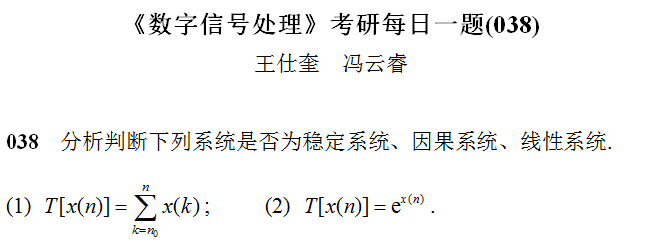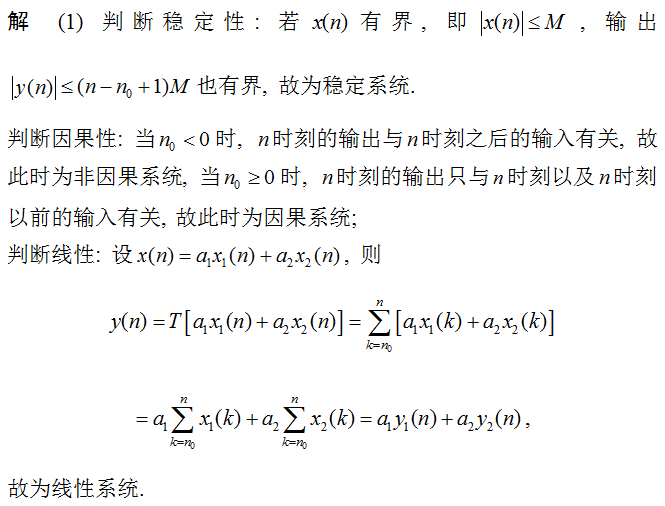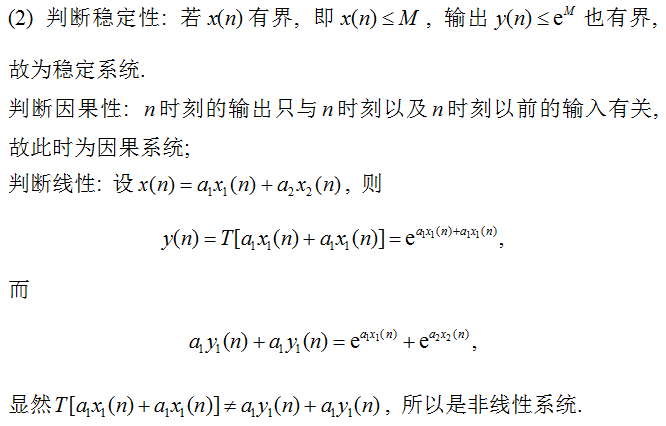展开全文• 一、判断某个系统是否是 “ 线性系统

# 一、判断某个系统是否是 “ 线性 “ 系统

系统 T T " 时不变系统 " , 输入序列输出序列 如下图所示 :

输入为 x 1 ( n ) x_1(n) 序列时 , 输出是 y 1 ( n ) y_1(n) 序列 ;

输入为 x 2 ( n ) x_2(n) 序列时 , 输出是 y 2 ( n ) y_2(n) 序列 ;

输入为 x 3 ( n ) x_3(n) 序列时 , 输出是 y 3 ( n ) y_3(n) 序列 ;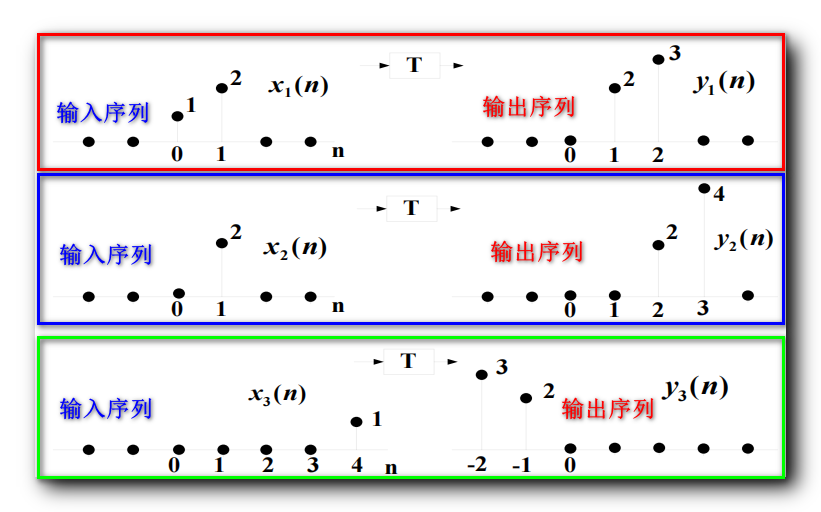判断上图中的系统 T T 是是否是 线性系统 ;

当系统为 T [ δ ( n ) ] T[\delta(n)] 时 , 输出是什么 ;

x 1 ( n ) = δ ( n ) + 2 δ ( n − 1 ) x_1(n) = \delta(n) + 2\delta(n - 1) , y 1 ( n ) = 2 δ ( n − 1 ) + 3 δ ( n − 2 ) y_1(n) = 2\delta(n - 1) + 3 \delta(n - 2)

x 2 ( n ) = 2 δ ( n − 1 ) x_2(n) = 2 \delta(n - 1) , y 2 ( n ) = 2 δ ( n − 2 ) + 4 δ ( n − 3 ) y_2(n) = 2\delta(n - 2) + 4 \delta(n - 3)

x 3 ( n ) = δ ( n − 4 ) x_3(n) = \delta(n - 4) , y 3 ( n ) = 2 δ ( n + 1 ) + 3 δ ( n + 2 ) y_3(n) = 2\delta(n + 1) + 3 \delta(n + 2)

x 1 ( n ) = x 2 ( n ) + x 3 ( n + 4 ) x_1(n) = x_2(n) + x_3(n + 4) , 令 x 1 ( n ) x_1(n) 中的 δ ( n ) \delta(n) 等于 x 3 ( n ) x_3(n) 中的 δ ( n − 4 ) \delta(n - 4) , 向左移 4 4 即可 ;

在该系统是 " 时不变 " 系统的前提下 , 如果 y 1 ( n ) = y 2 ( n ) + y 3 ( n + 4 ) y_1(n) = y_2(n) + y_3(n + 4) , 那么说明该系统是 " 线性 " 系统 ;

y 1 ( n ) = y 2 ( n ) + y 3 ( n + 4 ) y_1(n) = y_2(n) + y_3(n + 4)

y 2 ( n ) + y 3 ( n + 4 ) = 2 δ ( n − 2 ) + 4 δ ( n − 3 ) + 2 δ ( n + 5 ) + 3 δ ( n + 6 ) y_2(n) + y_3(n + 4) =2\delta(n - 2) + 4 \delta(n - 3) + 2\delta(n + 5) + 3 \delta(n + 6) , 明显不等于 y 1 ( n ) = 2 δ ( n − 1 ) + 3 δ ( n − 2 ) y_1(n) = 2\delta(n - 1) + 3 \delta(n - 2) ;

该系统 , 不是 " 线性 " 系统 ;

T [ δ ( n ) ] T[\delta(n)] 系统中 , 如果 输入是 δ ( n ) \delta(n) 序列 , 则对应的 " 变换 " 后的输出是 y 3 ( n + 4 ) = 2 δ ( n + 5 ) + 3 δ ( n + 6 ) y_3(n + 4) = 2\delta(n + 5) + 3 \delta(n + 6) , 得到如下公式 :
T [ δ ( n ) ] = 2 δ ( n + 5 ) + 3 δ ( n + 6 ) T[\delta(n)] = 2\delta(n + 5) + 3 \delta(n + 6)

展开全文数字信号处理
• 线性系统 顾名思义，满足线性性质的系统 它有三个特性： 齐次性 可加性 线性性 这里常见在选择题里让你判断 f1(.)f_{1}(.)f1​(.)代表的是激励，系统的响应不仅和它当前的状态相关，还和以前的状态相关的系统被...

今天我们说一说系统的分类

#### 线性系统

顾名思义，满足线性性质的系统
它有三个特性：

1. 齐次性
2. 可加性
3. 线性性
这里常见在选择题里让你判断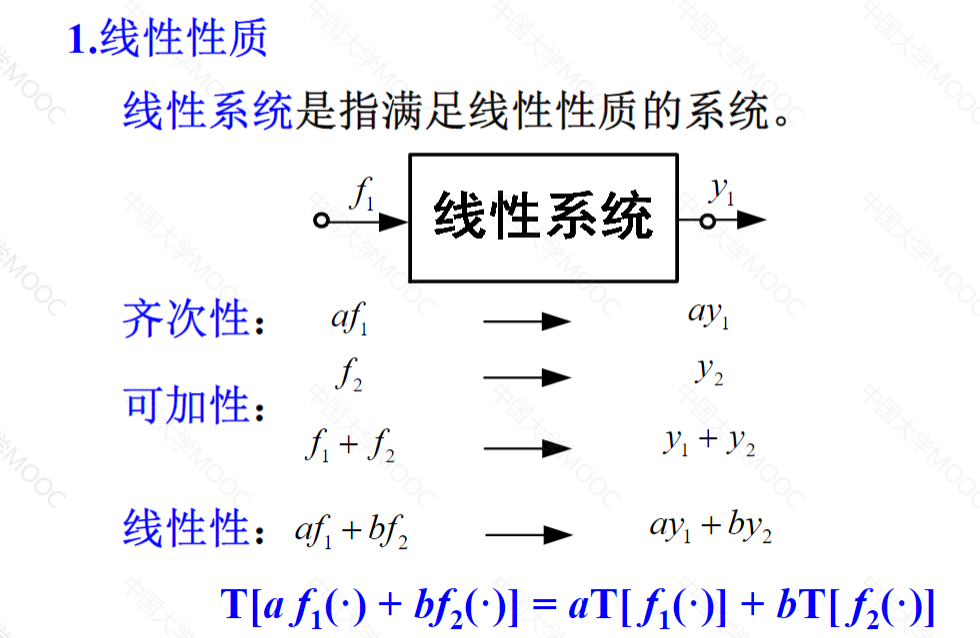f 1 ( . ) f_{1}(.) 代表的是激励，系统的响应不仅和它当前的状态相关，还和以前的状态相关的系统被称为记忆系统，电路与系统就是一家，这类电路通常包含电感与电容，反之则称为即时系统或无记忆系统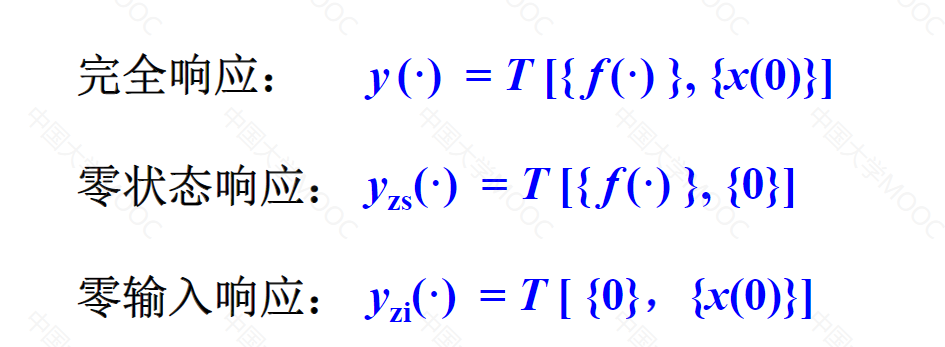全响应：由储能元件的初始储能和独立电源共同引起的响应，称为完全响应，简称全响应。
零输入响应：在没有外加激励时，仅由t = 0时刻的非零初始状态引起的响应。取决于初始状态和电路特性，这种响应随时间按指数规律衰减。
零状态响应：就是电路在零初始状态下（动态元件初始储能为零）由外施激励引起的响应

这三个响应后面会出答题，掌握规律，其实并不难

那么如何才能判断一个系统是不是线性的呢？
这就要用到刚才列举的三个响应的式子了

全响应=零输入+零状态

y ( t ) = y x ( t ) + y f ( t ) y(t)=y_x(t)+y_f(t)
拿到一个式子，首先看能不能把它分解为这两个子式，举个例子：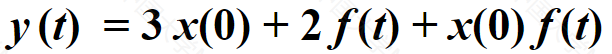显而易见， x ( 0 ) f ( t ) x(0)f(t) 是分不开的，只要分不开，就不满足分解性，它就不是线性的，我们再来看下面几个线性判别依据：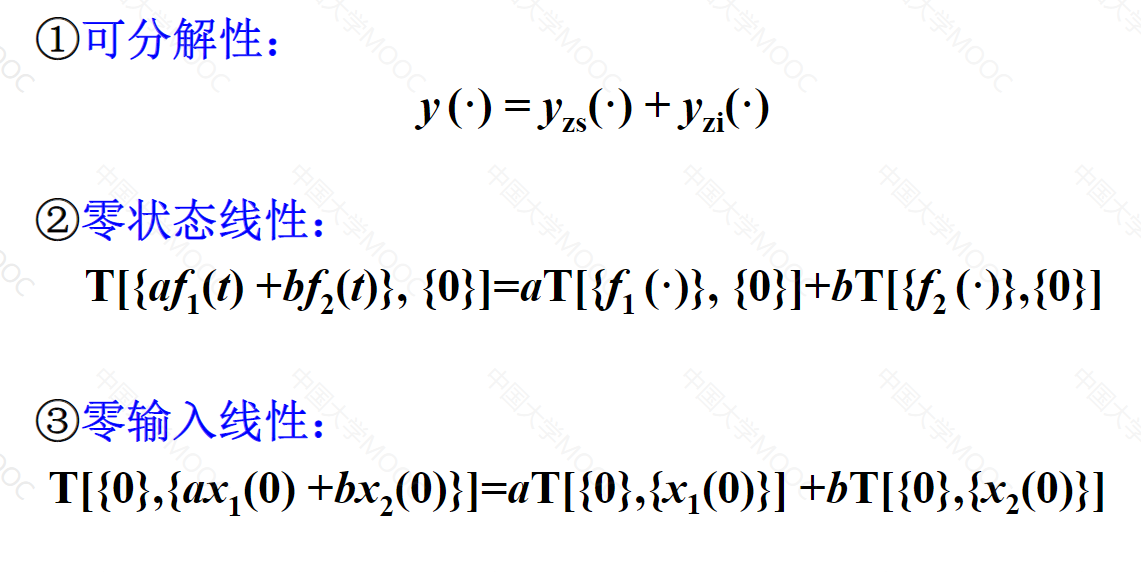再看一题：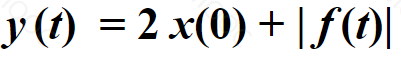这个我们要用到零状态线性来判断，就是 f ( t ) f(t) 前面有一个系数 a ( a ≠ 0 ) a(a\neq0) 但是这里的 f ( t ) f(t) 带了一个绝对值，所以你把a提到外面之后是不能保证a在绝对值符号里面时 f ( t ) f(t) 的值与原来相等，也可以理解看到 ∣ f ( t ) ∣ |f(t)| 这种带了个绝对值它十有八九不满足线性（当然，遇到复杂情况自己演算一下）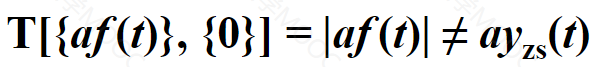最后一题：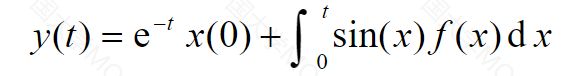判断一个系统是不是线性系统分三步走：
1.是否满足可分解性？可以看到0输入与0状态响应是分开的，故满足
2.是否满足0状态线性？
看是否满足这个条件：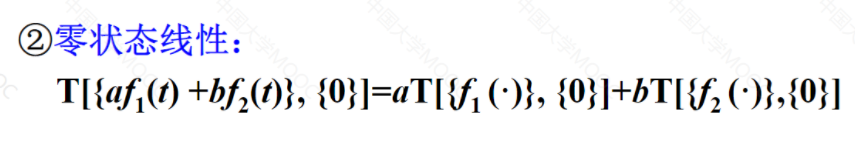就把前面的 a f 1 ( t ) + b f 2 ( t ) af_1(t)+bf_2(t) 带入 f ( x ) f(x)
得到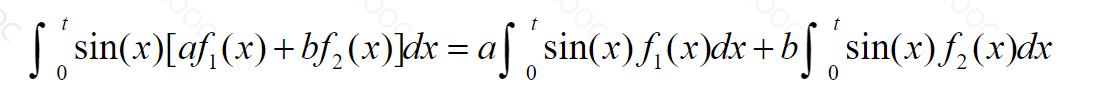满足了输入的线性组合等于输出的线性组合这一条件，即为满足了0状态线性

3.零输入线性
e − t x ( 0 ) e^{-t}x(0) 这一项代表的是0输入响应，因为它有 x ( 0 ) x(0) ，自变量是t，和判断0状态线性一样的道理，把 [ a f 1 ( x ) + b f 2 ( x ) ] [af_1(x)+bf_2(x)] 代入，得到：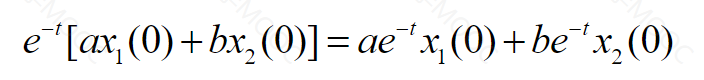也满足了输入线性组合等于输出线性组合这一条件。综上，这个系统是线性系统

展开全文数字信号处理
• 一、判断系统是否 " 非时变 "、 1、案例二、 ① 时不变系统概念、 ② 先变换后移位、 ③ 先移位后变换、 ④ 结论、数字信号处理
• 信号与线性系统· 吴大正 课后习题 全解 PDF文件
• 对于线性系统，我们有成熟的判断方法．然而，对于非线性系统则无统一的判别方法．Elliott在2005年给出了一个二阶双线性系统经过离散化后，可控性发生变化的例子．它表明一个系统在离散化前后，它的可控性可能会发生...
• 控制系统中的传感器、执行器和被控对象的故障检测与诊断往往是把执行器和被控对象视为一个整体来研究, 这不利于故障的决策与处理.... 最后以单输入单输出非线性系统为例进行仿真, 验证了该方法的有效性.</p>
• 一、判断系统是否 " 非时变 "、 1、案例二、 ① 时不变系统概念、 ② 先变换后移位、 ③ 先移位后变换、 ④ 结论、数字信号处理
• 判断系统线性时变因果方法PPT教案学习.pptx
• 线性系统理论中,渐近稳定性判据占有重妥的地位,目前常用的四种据 Routh-Hurwitz 4判断,连分式Hurwitz行列据和Lienard-Chipart判据 ,本文通过引入一个可实现的系统,揭示了四种-PJ据间的内在联系,给出了这四种荆据...
• 线性系统稳定性分析的MATLAB方法 ;线性系统稳定的充分必要条件 线性系统的稳定性仅取决于系统自身的固有特性而与外界条件无关 ;代数法;根轨迹法判断系统稳定性;奈奎斯特图法判断系统稳定性;波特图法判断系统稳定性;1...
• Matlab 在线性系统稳定性判断中的应用.rar
• 微分方程描述系统线性判断PPT学习教案.pptx
• Matlab 在线性系统稳定性判断中的应用.pdfmatlab
• 二、如何判断一个系统是线形还是非线性系统？ 如果从系统状态空间表达式来观察，线性系统和非线性系统最明显的区别方式就是线性系统符合叠加原理，而非线性系统不然。换句话说线性系统只有状态变量的一次项。高次、...
• 一、判断系统是否 " 非时变 "、 1、案例一、 ① 时不变系统、 ② 先变换后移位、 ③ 先移位后变换、 ④ 结论、数字信号处理
• ## 线性时不变因果系统的判断方法

千次阅读 多人点赞 2021-03-26 09:52:32
线性时不变因果电路的判断方法 在信号与系统的学习中 我们最常遇到的系统就是线性时不变因果系统的分析 下面我们来看一下如何判断线性 时不变 和因果的方法
• 根据Metzler矩阵定义,利用线性矩阵非负性约束给出了判断Metzler矩阵的充要条件,结合Drazin逆和矩阵拉直算子的相关性质,利用最小绝对差给出了一种新的判定连续正奇异系统正性的线性规划方法,同传统方法相比,本文方法...
• 一、" 线性常系数差分方程 " 与 " 线性时不变系统 " 关联、 二、根据 " 线性常系数差分方程 " 与 " 边界条件 " 确定系统是否是 " 线性时不变系统 " 方法、 1、线性时不变系统概念 ( 叠加性 | 不随着时间的变化而变化...数字信号处理
• 文章目录判别线性系统与非线性系统直观判别法线性系统与非线性系统的区别判别时变与时不变系统 判别线性系统与非线性系统 直观判别法 线性系统的表达式中只有状态变量的一次项，高次、三角函数以及常数项都没有，...
• 首先根据摄动法求解非线性微分方程的理论，论证GNAR模型与线性和弱非线性系统之间的本质联系，推导出GNAR模型系数与线性和非线性系统频率之间的解析关系；然后给出由GNAR模型系数和结构判断系统是否存在非线性及辨识...
• matlab非线性控制系统分析MATLAB与控制系统仿真实践 第16章 非线性控制系统分析 主要内容 原理要点 非线性系统概述 相平面法 描述平面法 原理要点 非线性系统的研究方法由于系统的复杂性和多样性而成为控制界的研究...
• 网络游戏-基于网络编码的内容分发系统线性相关性判断方法和装置.zip
• 用Brouwer不动点定理研究非线性自治离散控制系统和非自治离散控制系统的可观测性....M列满秩,且对每个i∈[h,h+N-2 ](i正整数), f(i,x(i))关于x(i)连续且有界,则非自治离散控制系统在第h阶段是局部可观测的.
• 线性系统 线性系统是指同时满足叠加性与均匀性（又称为其次性）的系统。...不满足叠加性和均匀性的系统即线性系统。 非线性系统线性系统：一个系统,如果其输出不与其输入成正比，则它是非线性的。从数学......